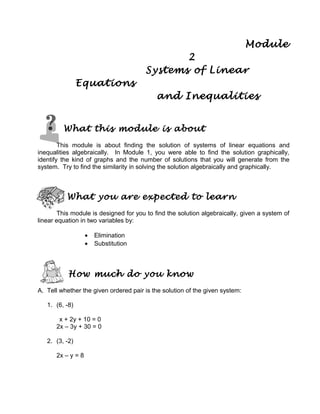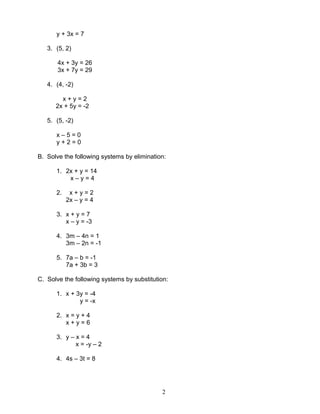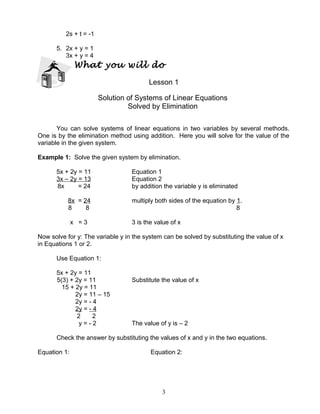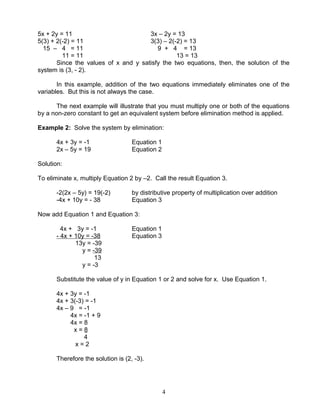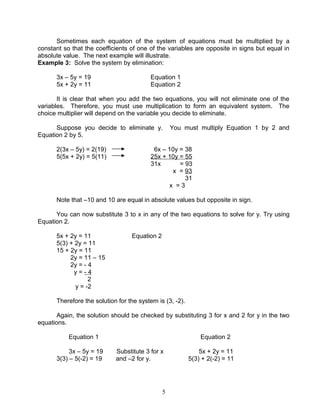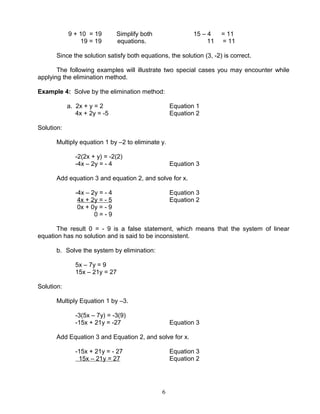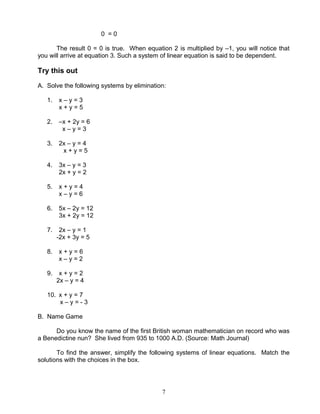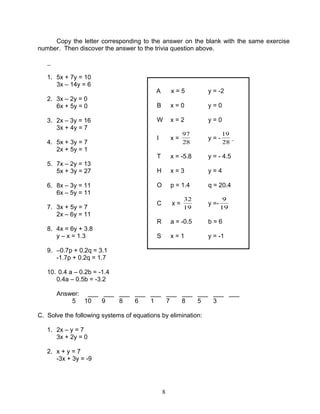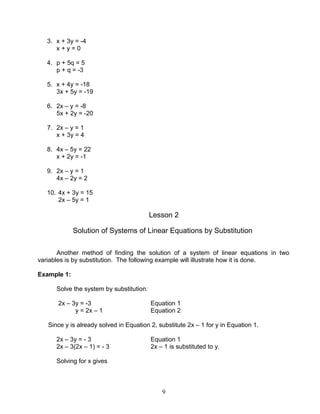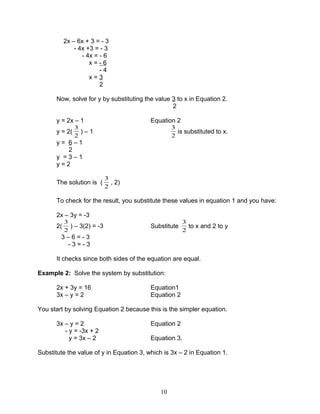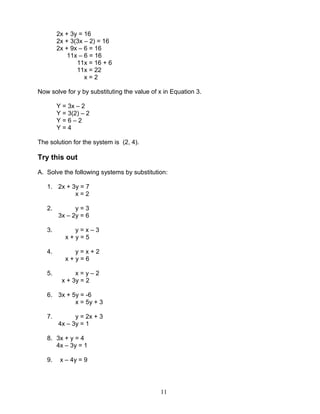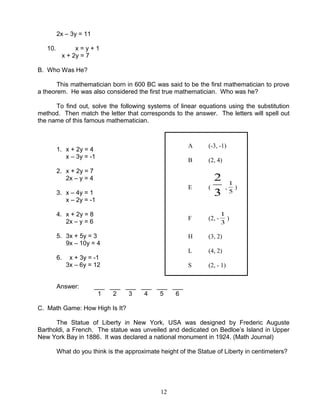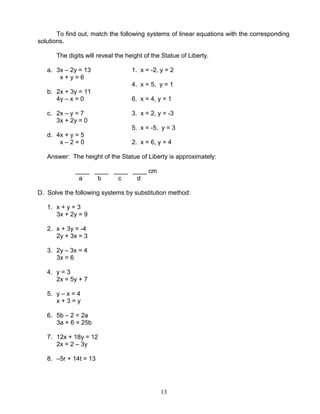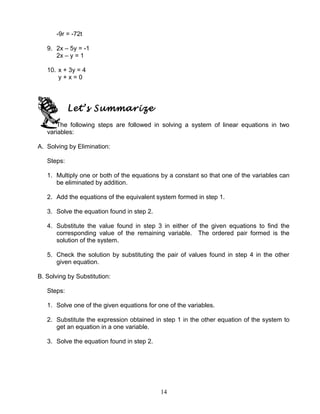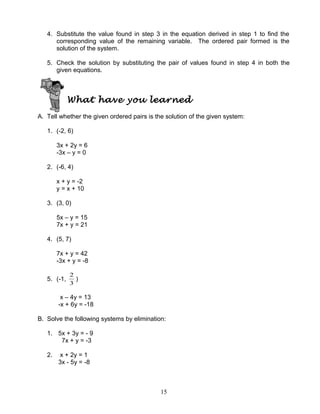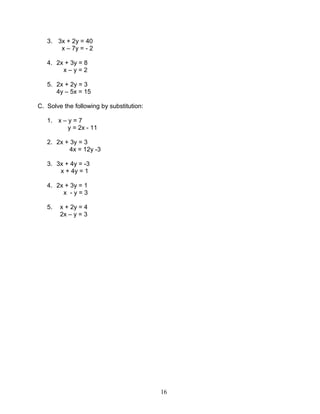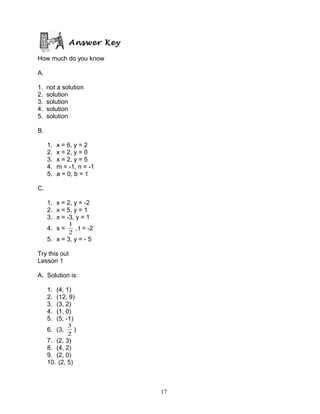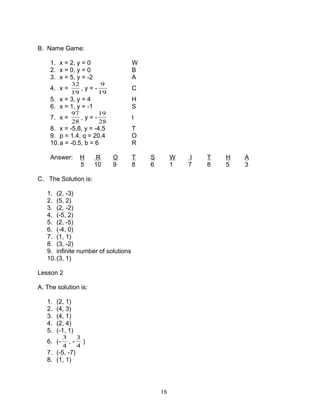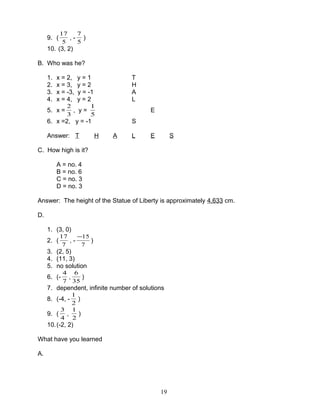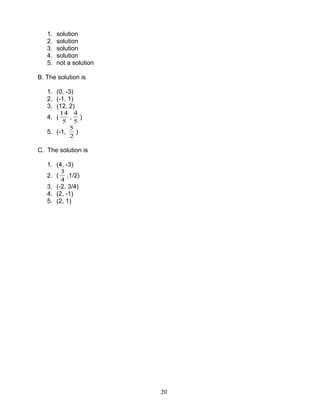Nächste SlideShare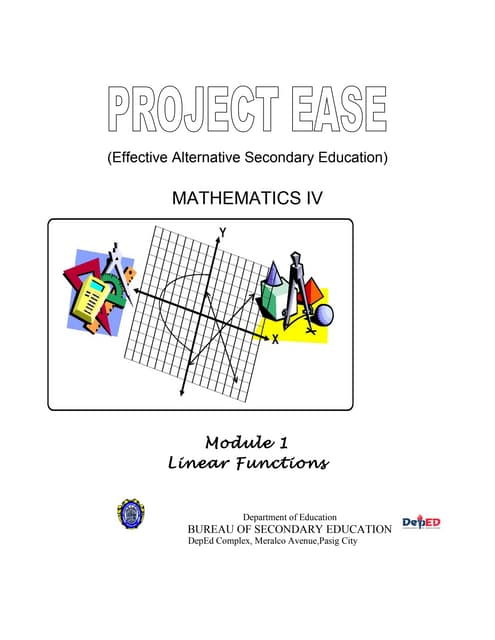Mathematics 8 Linear Functions
1 von 20Mathematics 8 Linear FunctionsJuan Miguel PaleroLinear Equations and Inequalities in One Variablemisey_margaretteMath 8 - Linear Inequalities in Two VariablesCarlo LunaLesson plan on factoring polynomial with common monomial factorLorie Jane LetadaMath10 q2 mod3of8_theorems on chords, arcs, central angles and inscribed angl...FahadOdin

### Mathematics 8 Systems of Linear Inequalities

1. Module 2 Systems of Linear Equations and Inequalities What this module is about This module is about finding the solution of systems of linear equations and inequalities algebraically. In Module 1, you were able to find the solution graphically, identify the kind of graphs and the number of solutions that you will generate from the system. Try to find the similarity in solving the solution algebraically and graphically. What you are expected to learn This module is designed for you to find the solution algebraically, given a system of linear equation in two variables by: • Elimination • Substitution How much do you know A. Tell whether the given ordered pair is the solution of the given system: 1. (6, -8) x + 2y + 10 = 0 2x – 3y + 30 = 0 2. (3, -2) 2x – y = 8
2. y + 3x = 7 3. (5, 2) 4x + 3y = 26 3x + 7y = 29 4. (4, -2) x + y = 2 2x + 5y = -2 5. (5, -2) x – 5 = 0 y + 2 = 0 B. Solve the following systems by elimination: 1. 2x + y = 14 x – y = 4 2. x + y = 2 2x – y = 4 3. x + y = 7 x – y = -3 4. 3m – 4n = 1 3m – 2n = -1 5. 7a – b = -1 7a + 3b = 3 C. Solve the following systems by substitution: 1. x + 3y = -4 y = -x 2. x = y + 4 x + y = 6 3. y – x = 4 x = -y – 2 4. 4s – 3t = 8 2
3. 2s + t = -1 5. 2x + y = 1 3x + y = 4 What you will do Lesson 1 Solution of Systems of Linear Equations Solved by Elimination You can solve systems of linear equations in two variables by several methods. One is by the elimination method using addition. Here you will solve for the value of the variable in the given system. Example 1: Solve the given system by elimination. 5x + 2y = 11 Equation 1 3x – 2y = 13 Equation 2 8x = 24 by addition the variable y is eliminated 8x = 24 multiply both sides of the equation by 1. 8 8 8 x = 3 3 is the value of x Now solve for y: The variable y in the system can be solved by substituting the value of x in Equations 1 or 2. Use Equation 1: 5x + 2y = 11 5(3) + 2y = 11 Substitute the value of x 15 + 2y = 11 2y = 11 – 15 2y = - 4 2y = - 4 2 2 y = - 2 The value of y is – 2 Check the answer by substituting the values of x and y in the two equations. Equation 1: Equation 2: 3
4. 5x + 2y = 11 3x – 2y = 13 5(3) + 2(-2) = 11 3(3) – 2(-2) = 13 15 – 4 = 11 9 + 4 = 13 11 = 11 13 = 13 Since the values of x and y satisfy the two equations, then, the solution of the system is (3, - 2). In this example, addition of the two equations immediately eliminates one of the variables. But this is not always the case. The next example will illustrate that you must multiply one or both of the equations by a non-zero constant to get an equivalent system before elimination method is applied. Example 2: Solve the system by elimination: 4x + 3y = -1 Equation 1 2x – 5y = 19 Equation 2 Solution: To eliminate x, multiply Equation 2 by –2. Call the result Equation 3. -2(2x – 5y) = 19(-2) by distributive property of multiplication over addition -4x + 10y = - 38 Equation 3 Now add Equation 1 and Equation 3: 4x + 3y = -1 Equation 1 - 4x + 10y = -38 Equation 3 13y = -39 y = -39 13 y = -3 Substitute the value of y in Equation 1 or 2 and solve for x. Use Equation 1. 4x + 3y = -1 4x + 3(-3) = -1 4x – 9 = -1 4x = -1 + 9 4x = 8 x = 8 4 x = 2 Therefore the solution is (2, -3). 4
5. Sometimes each equation of the system of equations must be multiplied by a constant so that the coefficients of one of the variables are opposite in signs but equal in absolute value. The next example will illustrate. Example 3: Solve the system by elimination: 3x – 5y = 19 Equation 1 5x + 2y = 11 Equation 2 It is clear that when you add the two equations, you will not eliminate one of the variables. Therefore, you must use multiplication to form an equivalent system. The choice multiplier will depend on the variable you decide to eliminate. Suppose you decide to eliminate y. You must multiply Equation 1 by 2 and Equation 2 by 5. 2(3x – 5y) = 2(19) 6x – 10y = 38 5(5x + 2y) = 5(11) 25x + 10y = 55 31x = 93 x = 93 31 x = 3 Note that –10 and 10 are equal in absolute values but opposite in sign. You can now substitute 3 to x in any of the two equations to solve for y. Try using Equation 2. 5x + 2y = 11 Equation 2 5(3) + 2y = 11 15 + 2y = 11 2y = 11 – 15 2y = - 4 y = - 4 2 y = -2 Therefore the solution for the system is (3, -2). Again, the solution should be checked by substituting 3 for x and 2 for y in the two equations. Equation 1 Equation 2 3x – 5y = 19 Substitute 3 for x 5x + 2y = 11 3(3) – 5(-2) = 19 and –2 for y. 5(3) + 2(-2) = 11 5
6. 9 + 10 = 19 Simplify both 15 – 4 = 11 19 = 19 equations. 11 = 11 Since the solution satisfy both equations, the solution (3, -2) is correct. The following examples will illustrate two special cases you may encounter while applying the elimination method. Example 4: Solve by the elimination method: a. 2x + y = 2 Equation 1 4x + 2y = -5 Equation 2 Solution: Multiply equation 1 by –2 to eliminate y. -2(2x + y) = -2(2) -4x – 2y = - 4 Equation 3 Add equation 3 and equation 2, and solve for x. -4x – 2y = - 4 Equation 3 4x + 2y = - 5 Equation 2 0x + 0y = - 9 0 = - 9 The result 0 = - 9 is a false statement, which means that the system of linear equation has no solution and is said to be inconsistent. b. Solve the system by elimination: 5x – 7y = 9 15x – 21y = 27 Solution: Multiply Equation 1 by –3. -3(5x – 7y) = -3(9) -15x + 21y = -27 Equation 3 Add Equation 3 and Equation 2, and solve for x. -15x + 21y = - 27 Equation 3 15x – 21y = 27 Equation 2 6
7. 0 = 0 The result 0 = 0 is true. When equation 2 is multiplied by –1, you will notice that you will arrive at equation 3. Such a system of linear equation is said to be dependent. Try this out A. Solve the following systems by elimination: 1. x – y = 3 x + y = 5 2. –x + 2y = 6 x – y = 3 3. 2x – y = 4 x + y = 5 4. 3x – y = 3 2x + y = 2 5. x + y = 4 x – y = 6 6. 5x – 2y = 12 3x + 2y = 12 7. 2x – y = 1 -2x + 3y = 5 8. x + y = 6 x – y = 2 9. x + y = 2 2x – y = 4 10. x + y = 7 x – y = - 3 B. Name Game Do you know the name of the first British woman mathematician on record who was a Benedictine nun? She lived from 935 to 1000 A.D. (Source: Math Journal) To find the answer, simplify the following systems of linear equations. Match the solutions with the choices in the box. 7
8. Copy the letter corresponding to the answer on the blank with the same exercise number. Then discover the answer to the trivia question above. 1. 5x + 7y = 10 3x – 14y = 6 2. 3x – 2y = 0 6x + 5y = 0 3. 2x – 3y = 16 3x + 4y = 7 4. 5x + 3y = 7 2x + 5y = 1 5. 7x – 2y = 13 5x + 3y = 27 6. 8x – 3y = 11 6x – 5y = 11 7. 3x + 5y = 7 2x – 6y = 11 8. 4x = 6y + 3.8 y – x = 1.3 9. –0.7p + 0.2q = 3.1 -1.7p + 0.2q = 1.7 10. 0.4 a – 0.2b = -1.4 0.4a – 0.5b = -3.2 Answer: ___ ___ ___ ___ ___ ___ ___ ___ ___ ___ 5 10 9 8 6 1 7 8 5 3 C. Solve the following systems of equations by elimination: 1. 2x – y = 7 3x + 2y = 0 2. x + y = 7 -3x + 3y = -9 8 A x = 5 y = -2 B x = 0 y = 0 W x = 2 y = 0 I x = 28 97 y = - 28 19 T x = -5.8 y = - 4.5 H x = 3 y = 4 O p = 1.4 q = 20.4 C x = 19 32 y =- 19 9 R a = -0.5 b = 6 S x = 1 y = -1
9. 3. x + 3y = -4 x + y = 0 4. p + 5q = 5 p + q = -3 5. x + 4y = -18 3x + 5y = -19 6. 2x – y = -8 5x + 2y = -20 7. 2x – y = 1 x + 3y = 4 8. 4x – 5y = 22 x + 2y = -1 9. 2x – y = 1 4x – 2y = 2 10. 4x + 3y = 15 2x – 5y = 1 Lesson 2 Solution of Systems of Linear Equations by Substitution Another method of finding the solution of a system of linear equations in two variables is by substitution. The following example will illustrate how it is done. Example 1: Solve the system by substitution: 2x – 3y = -3 Equation 1 y = 2x – 1 Equation 2 Since y is already solved in Equation 2, substitute 2x – 1 for y in Equation 1. 2x – 3y = - 3 Equation 1 2x – 3(2x – 1) = - 3 2x – 1 is substituted to y. Solving for x gives 9
10. 2x – 6x + 3 = - 3 - 4x +3 = - 3 - 4x = - 6 x = - 6 - 4 x = 3 2 Now, solve for y by substituting the value 3 to x in Equation 2. 2 y = 2x – 1 Equation 2 y = 2( 2 3 ) – 1 2 3 is substituted to x. y = 6 – 1 2 y = 3 – 1 y = 2 The solution is ( 2 3 , 2) To check for the result, you substitute these values in equation 1 and you have: 2x – 3y = -3 2( 2 3 ) – 3(2) = -3 Substitute 2 3 to x and 2 to y 3 – 6 = - 3 - 3 = - 3 It checks since both sides of the equation are equal. Example 2: Solve the system by substitution: 2x + 3y = 16 Equation1 3x – y = 2 Equation 2 You start by solving Equation 2 because this is the simpler equation. 3x – y = 2 Equation 2 - y = -3x + 2 y = 3x – 2 Equation 3. Substitute the value of y in Equation 3, which is 3x – 2 in Equation 1. 10
11. 2x + 3y = 16 2x + 3(3x – 2) = 16 2x + 9x – 6 = 16 11x – 6 = 16 11x = 16 + 6 11x = 22 x = 2 Now solve for y by substituting the value of x in Equation 3. Y = 3x – 2 Y = 3(2) – 2 Y = 6 – 2 Y = 4 The solution for the system is (2, 4). Try this out A. Solve the following systems by substitution: 1. 2x + 3y = 7 x = 2 2. y = 3 3x – 2y = 6 3. y = x – 3 x + y = 5 4. y = x + 2 x + y = 6 5. x = y – 2 x + 3y = 2 6. 3x + 5y = -6 x = 5y + 3 7. y = 2x + 3 4x – 3y = 1 8. 3x + y = 4 4x – 3y = 1 9. x – 4y = 9 11
12. 2x – 3y = 11 10. x = y + 1 x + 2y = 7 B. Who Was He? This mathematician born in 600 BC was said to be the first mathematician to prove a theorem. He was also considered the first true mathematician. Who was he? To find out, solve the following systems of linear equations using the substitution method. Then match the letter that corresponds to the answer. The letters will spell out the name of this famous mathematician. 1. x + 2y = 4 x – 3y = -1 2. x + 2y = 7 2x – y = 4 3. x – 4y = 1 x – 2y = -1 4. x + 2y = 8 2x – y = 6 5. 3x + 5y = 3 9x – 10y = 4 6. x + 3y = -1 3x – 6y = 12 Answer: ___ ___ ___ ___ ___ ___ 1 2 3 4 5 6 C. Math Game: How High Is It? The Statue of Liberty in New York, USA was designed by Frederic Auguste Bartholdi, a French. The statue was unveiled and dedicated on Bedloe’s Island in Upper New York Bay in 1886. It was declared a national monument in 1924. (Math Journal) What do you think is the approximate height of the Statue of Liberty in centimeters? 12 A (-3, -1) B (2, 4) E ( 3 2 , 5 1 ) F (2, - 3 1 ) H (3, 2) L (4, 2) S (2, - 1)
13. To find out, match the following systems of linear equations with the corresponding solutions. The digits will reveal the height of the Statue of Liberty. a. 3x – 2y = 13 1. x = -2, y = 2 x + y = 6 4. x = 5, y = 1 b. 2x + 3y = 11 4y – x = 0 6. x = 4, y = 1 c. 2x – y = 7 3. x = 2, y = -3 3x + 2y = 0 5. x = -5, y = 3 d. 4x + y = 5 x – 2 = 0 2. x = 6, y = 4 Answer: The height of the Statue of Liberty is approximately: ____ ____ ____ ____ cm a b c d D. Solve the following systems by substitution method: 1. x + y = 3 3x + 2y = 9 2. x + 3y = -4 2y + 3x = 3 3. 2y – 3x = 4 3x = 6 4. y = 3 2x = 5y + 7 5. y – x = 4 x + 3 = y 6. 5b – 2 = 2a 3a + 6 = 25b 7. 12x + 18y = 12 2x = 2 – 3y 8. –5r + 14t = 13 13
14. -9r = -72t 9. 2x – 5y = -1 2x – y = 1 10. x + 3y = 4 y + x = 0 Let’s Summarize The following steps are followed in solving a system of linear equations in two variables: A. Solving by Elimination: Steps: 1. Multiply one or both of the equations by a constant so that one of the variables can be eliminated by addition. 2. Add the equations of the equivalent system formed in step 1. 3. Solve the equation found in step 2. 4. Substitute the value found in step 3 in either of the given equations to find the corresponding value of the remaining variable. The ordered pair formed is the solution of the system. 5. Check the solution by substituting the pair of values found in step 4 in the other given equation. B. Solving by Substitution: Steps: 1. Solve one of the given equations for one of the variables. 2. Substitute the expression obtained in step 1 in the other equation of the system to get an equation in a one variable. 3. Solve the equation found in step 2. 14
15. 4. Substitute the value found in step 3 in the equation derived in step 1 to find the corresponding value of the remaining variable. The ordered pair formed is the solution of the system. 5. Check the solution by substituting the pair of values found in step 4 in both the given equations. What have you learned A. Tell whether the given ordered pairs is the solution of the given system: 1. (-2, 6) 3x + 2y = 6 -3x – y = 0 2. (-6, 4) x + y = -2 y = x + 10 3. (3, 0) 5x – y = 15 7x + y = 21 4. (5, 7) 7x + y = 42 -3x + y = -8 5. (-1, 3 2 ) x – 4y = 13 -x + 6y = -18 B. Solve the following systems by elimination: 1. 5x + 3y = - 9 7x + y = -3 2. x + 2y = 1 3x - 5y = -8 15
16. 3. 3x + 2y = 40 x – 7y = - 2 4. 2x + 3y = 8 x – y = 2 5. 2x + 2y = 3 4y – 5x = 15 C. Solve the following by substitution: 1. x – y = 7 y = 2x - 11 2. 2x + 3y = 3 4x = 12y -3 3. 3x + 4y = -3 x + 4y = 1 4. 2x + 3y = 1 x - y = 3 5. x + 2y = 4 2x – y = 3 16
17. Answer Key How much do you know A. 1. not a solution 2. solution 3. solution 4. solution 5. solution B. 1. x = 6, y = 2 2. x = 2, y = 0 3. x = 2, y = 5 4. m = -1, n = -1 5. a = 0, b = 1 C. 1. x = 2, y = -2 2. x = 5, y = 1 3. x = -3, y = 1 4. s = 2 1 , t = -2 5. x = 3, y = - 5 Try this out Lesson 1 A. Solution is: 1. (4, 1) 2. (12, 9) 3. (3, 2) 4. (1, 0) 5. (5, -1) 6. (3, 2 3 ) 7. (2, 3) 8. (4, 2) 9. (2, 0) 10. (2, 5) 17
18. B. Name Game: 1. x = 2, y = 0 W 2. x = 0, y = 0 B 3. x = 5, y = -2 A 4. x = 19 32 , y = - 19 9 C 5. x = 3, y = 4 H 6. x = 1, y = -1 S 7. x = 28 97 , y = - 28 19 I 8. x = -5.8, y = -4.5 T 9. p = 1.4, q = 20.4 O 10.a = -0.5, b = 6 R Answer: H R O T S W I T H A 5 10 9 8 6 1 7 8 5 3 C. The Solution is: 1. (2, -3) 2. (5, 2) 3. (2, -2) 4. (-5, 2) 5. (2, -5) 6. (-4, 0) 7. (1, 1) 8. (3, -2) 9. infinite number of solutions 10.(3, 1) Lesson 2 A. The solution is: 1. (2, 1) 2. (4, 3) 3. (4, 1) 4. (2, 4) 5. (-1, 1) 6. (- 4 3 , - 4 3 ) 7. (-5, -7) 8. (1, 1) 18
19. 9. ( 5 17 , - 5 7 ) 10. (3, 2) B. Who was he? 1. x = 2, y = 1 T 2. x = 3, y = 2 H 3. x = -3, y = -1 A 4. x = 4, y = 2 L 5. x = 3 2 , y = 5 1 E 6. x =2, y = -1 S Answer: T H A L E S C. How high is it? A = no. 4 B = no. 6 C = no. 3 D = no. 3 Answer: The height of the Statue of Liberty is approximately 4,633 cm. D. 1. (3, 0) 2. ( 7 17 , - 7 15− ) 3. (2, 5) 4. (11, 3) 5. no solution 6. (- 7 4 , 35 6 ) 7. dependent, infinite number of solutions 8. (-4, - 2 1 ) 9. ( 4 3 , 2 1 ) 10.(-2, 2) What have you learned A. 19
20. 1. solution 2. solution 3. solution 4. solution 5. not a solution B. The solution is 1. (0, -3) 2. (-1, 1) 3. (12, 2) 4. ( 5 14 , 5 4 ) 5. (-1, 2 5 ) C. The solution is 1. (4, -3) 2. ( 4 3 ,1/2) 3. (-2, 3/4) 4. (2, -1) 5. (2, 1) 20